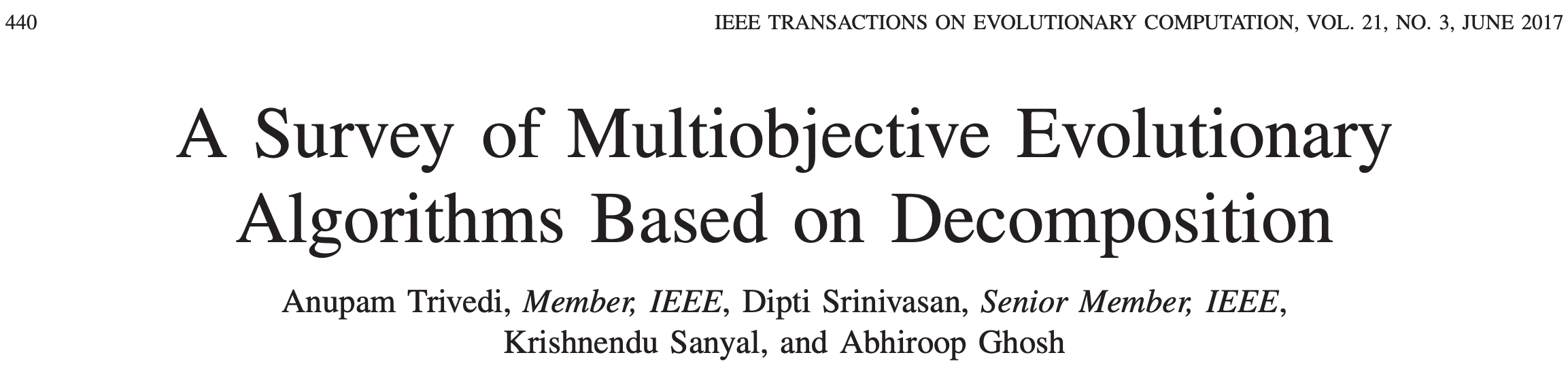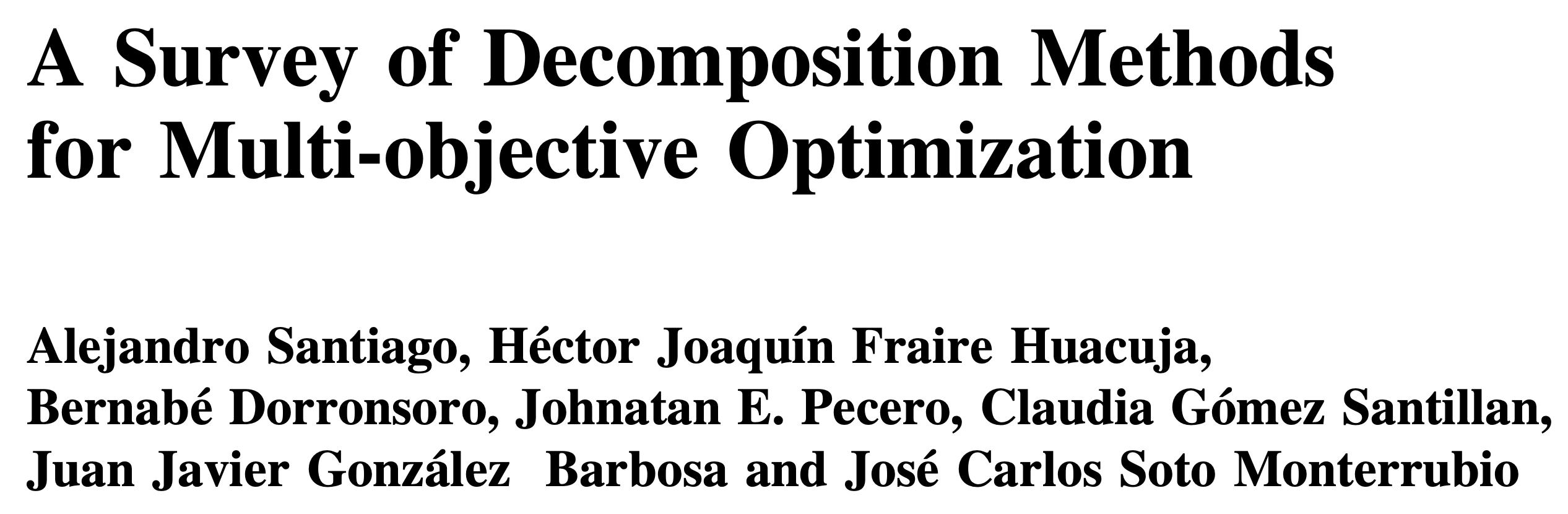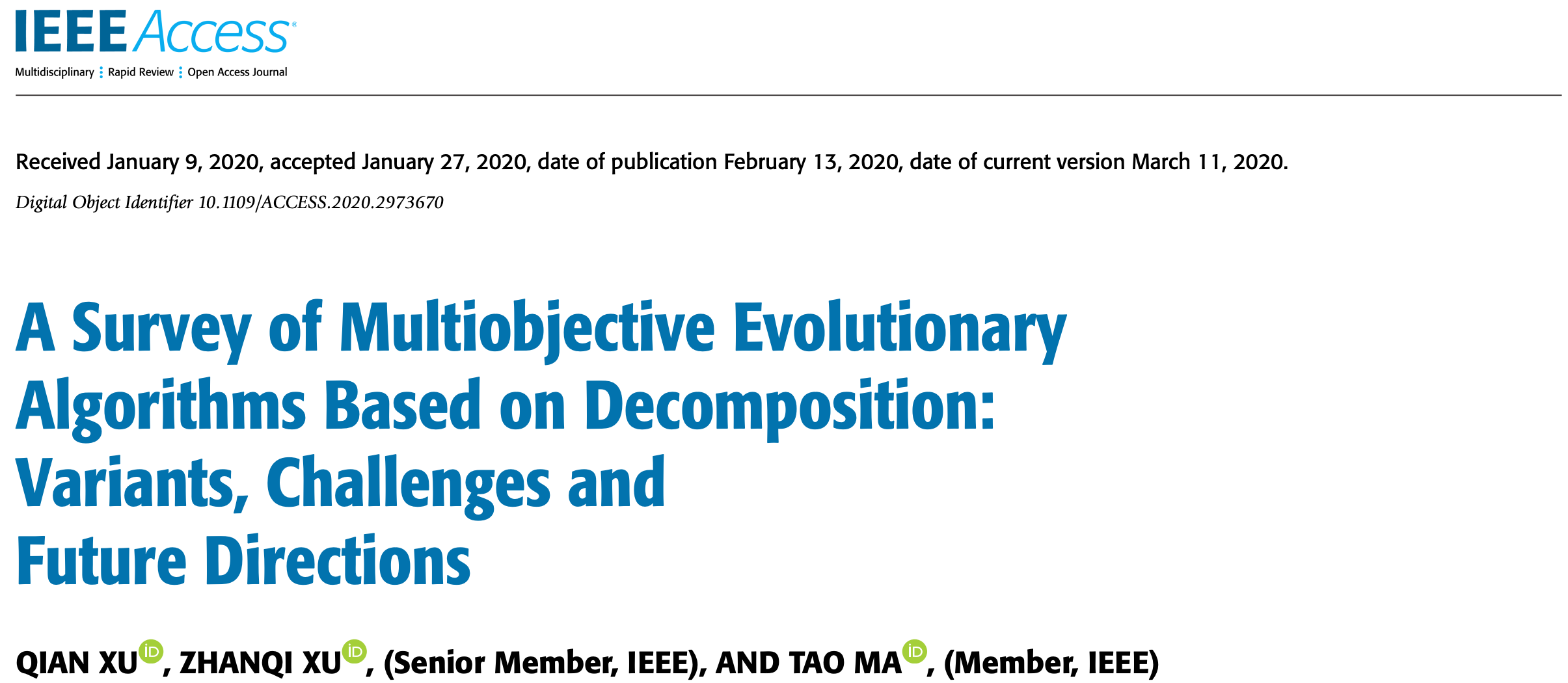MOEA/D: Multi-Objective Evolutionary Algorithm Based on Decomposition

CVPR Tutorial

June 19, 2023

## MOEA/D=Decomposition + Collaboration

• Decompose the task of approximating the PF into $N$ subproblems. Each subproblem can be single-objective or multiobjective.

• Collaboration (from swarm Intelligence and evolutionary computation)
• $N$ agents (procedures) are used. Each agent is for a different subproblem.
• These $N$ subproblems are related to one another. $N$ agents can solve these subproblems in a collaborative manner.

## Major Issues

• Problem decomposition and neighborhood structure.
• Search method used by each agent: memory and reproduction operators.
• Collaboration: mating selection and replacement.

## Problem Decomposition

Weighted Sum Approach

• $\min g^{ws}(x|\lambda) = \lambda_1f_1(x) + \lambda_2f_2(x)$ where $\lambda_1 + \lambda_2 = 1$ and $\lambda_1, \lambda_2 \geq 0$.
Works only for convex pareto-front.
Approximation of the Pareto Front $\Rightarrow$ $N$ single objective optimization problems.

## Tchebycheff Approach

Optimization Problem
\begin{aligned} \min \text{ } &g^T(\mathbf{x} | \lambda, \mathbf{z}^{*}) \\ g^T(\mathbf{x} | \lambda, \mathbf{z}^{*}) &= \max\{ \lambda_1 |f_1(\mathbf{x}) - z_1^{*}|, \lambda_2| f_2(\mathbf{x}) - z_2^{*}|\} \\ \mathbf{z}^{*} &= (z_1^*, z_2^*) \text{ is a Utopian point. } \\ & z_1^{*} < \min f_1 \text{ and } z_2^{*} < \min f_2 \end{aligned}

• For any Pareto optimal solution $\mathbf{x}^{*}$, there is a $\lambda$ such that $\mathbf{x}^{*}$ is optimal to the above problem.

• $g^T(\mathbf{x},\lambda)$ is not smooth w.r.t. $\mathbf{x}$,$\lambda$.

## Weighted $L_p$ Approach

Optimization Problem
\begin{equation} \begin{aligned} \min \text{ } &g^T(\mathbf{x} | \lambda, \mathbf{z}^{*}) \\ \text{ where } & g^T(\mathbf{x} | \lambda, \mathbf{z}^{*}) = \left(\lambda_1| f_1(\mathbf{x}) - z_1^{*}|^p + \lambda_2| f_2(\mathbf{x}) - z_2^{*}|^p\right)^{\frac{1}{p}} \\ \mathbf{z}^{*} &= (z_1^*, z_2^*) \text{ is a Utopian point.} \nonumber \end{aligned} \end{equation}
• $p=1:$ weighted sum
• $p=\infty:$ Tchebycheff

## Neighboring Subproblems

• Neighborhood: measure the relationship among the subtasks
• Two subproblems are neighbors if their weight vectors are close.
• Neighbouring subproblems should have similar objective functions and thus similar optimal solutions with high probability.
• Many different ways for defining neighborhood structure.

## Decomposition, Neighborhood and Memory

• At each generation, each agent does the following:
• Mating selection: obtain the current solutions of some neighbours (collaboration).
• Reproduction: generate a new solution by applying reproduction operators on its own solution and borrowed solutions.
• Replacement:
• Replace its old solution by the new one if the new one is better than old one for its objective.
• Pass the new solution on to some of its neighbours, each of them replaces its old solution by this new one if it is better for its objective (collaboration, neighbourhood).

## Simulation Results

• No of subproblems: 595, Reproduction operators: DE+Mutation
• No of generation: 500
• Test instance:
• \begin{aligned} f_1 &= cos(0.5x_1\pi)cos(0.5x_x\pi) + \frac{2}{|J_1|}\sum_{j\in J_1}(x_j - 2x_2sin(2\pi x_1 + \frac{j\pi}{n}))^2 \\ f_2 &= cos(0.5x_1\pi)sin(0.5x_x\pi) + \frac{2}{|J_2|}\sum_{j\in J_1}(x_j - 2x_2sin(2\pi x_1 + \frac{j\pi}{n}))^2 \\ f_3 &= sin(0.5x_1\pi) + \frac{2}{|J_3|}\sum_{j\in J_3}(x_j - 2x_2sin(2\pi x_1 + \frac{j\pi}{n}))^2 \\ \end{aligned}
• $J_1 = {j|3\leq j \leq n, \text{ and } j-1 \text{ is a multiplication of 3}}$,
• $J_2 = {j|3\leq j \leq n, \text{ and } j-2 \text{ is a multiplication of 3}}$,
• $J_3 = {j|3\leq j \leq n, \text{ and } j \text{ is a multiplication of 3}}$
• Pareto Surface: $x_j = 2x_2\sin(2\pi x_1 + \frac{j\pi}{n}), j=3,\dots,n$
Very hard and the optimal solution set is very complicated.

## Dynamic Resource Allocation

• Different subproblems (agents) require different amounts of computational resources.

• Each subproblem (agent) has a utility value, which measures the likelihood of further improvement: $Utility = \frac{\text{The amount of improvement obtained}}{\text{The amount of computational resources used}}$

• At each generation, a small number of agents are selected based on utility values and receive computational resources.

## Simulation Results

• Test instance:
• \begin{aligned} f_1 &= x_1 + \frac{2}{|J_1|}\sum_{j \in J_1}\left(x_j - 0.8x_1\cos\left(6\pi x_1 + \frac{j\pi}{n}\right)\right)^2 \\ f_2 &= 1 - \sqrt{x}_1 + \frac{2}{|J_2|}\sum_{j\in J_2}\left(x_j - 0.8x_1\sin\left(6\pi x_1 + \frac{j\pi}{n}\right)\right)^2\\ \end{aligned} Pareto Surface: $x_j = \begin{cases} 0.8x_1\cos\left(6\pi x_1 + \frac{j\pi}{n}\right) & j \in J_1 \\ 0.8x_1\sin\left(6\pi x_1 + \frac{j\pi}{n}\right) & j \in J_2 \end{cases}$
MOEA/D

• Diversity among subproblems leads to diversity among $\{\mathbf{x}^1, \mathbf{x}^2,\dots,\mathbf{x}^N\}$

• MOEA/D has a well-organized memory.

• Each agent can adopt other search methods such as heuristics (e.g., EDA, ACO and tabu search) or gradient based search.

• Agents can be divided into several groups. Each group share some common info such as probability models.

## Penalty based boundary interaction (PBI) (Zhang & Li 2007)

Optimization Problem
\begin{aligned} \min \text{ } &g^T(\mathbf{x} | \lambda, \mathbf{z}^{*}) = d_1 + \theta d_2 \\ s.t. & x \in \Omega \end{aligned}
• $\mathbf{z}^*,\lambda$ define a line direction.
• $\theta > 0$ is the penalty parameter
• $\theta \rightarrow \infty \Rightarrow \text{PBI} \rightarrow$ NBI (Normal boundary Interaction) (Das, 1998).
• Different Pareto optimal solutions can be obtained by using different direction lines.

## Penalty based boundary interaction (PBI) (Zhang & Li 2007)

• Diversity and Convergence:
Optimization Problem
\begin{aligned} \min \text{ } &g^T(\mathbf{x} | \lambda, \mathbf{z}^{*}) = d_1 + \theta d_2 \\ s.t. \text{ } & x \in \Omega \end{aligned}
• $d_1$: convergence
• $d_2$: diversity
• It has been used in EMO algorithm design such as in:
• I-DBEA (2015)
• MOEA/D-DU (2016)
• $\theta$-DEA (2016)

## Penalty based boundary interaction (PBI) (Zhang & Li 2007)

• Other Variants:
Optimization Problem
\begin{aligned} \min \text{ } &g^T(\mathbf{x} | \lambda, \mathbf{z}^{*}) = d_1 + \theta d_2 \\ s.t. \text{ } & x \in \Omega \end{aligned}
• $d_2$ can be replace by $\alpha$ (Chen et al, 2016)
• $g$ can be replaced by other functions (Ishibuchi et al, 2016, Chen et al 2016)
• NSGA-III = Pareto dominance (for convergence) + decomposition ($d_2$ for diversity) (Deb et al, 2013).

## Inverted PBI (IPBI, Sato 2014)

Optimization Problem
$\max g^{IPBI}(x|\lambda, z^*) = d_1 - \theta d_2$
• $z^+$ should be above the PF (nadir point)

• Difference between PBI and IPBI:
• When PF=ABC, some different subproblems in IPBI have the same optimal solutions.
• When PF=DEF, some different subproblems in PBI have the same optimal solutions.

## Constrained Decomposition

• Single objective decomposition (Wang et al, 2015)
• Optimization Problem
\begin{aligned} \min g(x) &= aggregation(f_1, f_2) \\ s.t. \text{ } & F(x) \in \Omega_i \end{aligned}
• Good for diversity

## MOEA/D for Expensive Multiobjective Optimization

• Expensive opt: function evaluation = expensive computer/physical experiments.
• The computational budget could be very limited, say, 200 function evaluations.
• Efficient Global Optimization (Bayesian Optimization) for single optimization problem do the following iteration:
• Using the evaluated solutions, build a probabilistic model $p(f│x)$ for objective $f(x)$.
• Use $p(f|x)$ to define acquisition function $\phi(x)$ (which measures the merits of figure of evaluation of objective $f$ at $x$.
• Maximize $\phi(x)$ to obtain $\bar{x}$, evaluate it.

## Experimental Results

• Experiments:
• ZDT3: 2 objectives and 6 decision variables.
• Computational budget: 150 function evaluations
MOEA/D-EGO (Zhang et al., 2009)

## PLS/D for Discrete Optimization

• Decompose the objective space into a number of sub-regions.
• Each subregion i is associated with an aggregated objective function: $\text{e.g., } g_{ws}(x, w_i) = 0.4f_1(x) + 0.6f_2(x)$
• Agent i searches Subregion i by using a PLS guided by $g_{ws}(x, w_i)$.

• J. Shi, Q. Zhang, J Sun, PLS/D, IEEE Trans on Cybernetics, 2019

## Experimental Results

• Test problem: multiobjective Unconstraint Binary Quadratic Programming
• Reproduction operators: Pareto local search (PLS)
• No of variables = 200. No. of objectives: 2 and 3
• No. of regions = 11 (for 2-obj) and 66 (for 3-obj)
classic PLS (2-obj)
PPLS/D (2-obj)
classic PLS (3-obj)
PPLS/D (3-obj)

• PPLS/D: Parallel Pareto Local Search Based on Decomposition, IEEE Transactions on Cybernetics, 2020

## ResourcesMOEA/D Website

## Survey Papers on MOEA/D## Survey Papers on MOEA/D## Conclusions

• MOEA/D= Decomposition + Collaboration, a methodology for multiobjective optimization.

• Key design issues: decomposition, search method for each agent, collaboration.

• Different single optimization methods can be readily used in MOEA/D framework.

• Multiobjective optimization methods can also be integrated in MOEA/D framework.